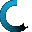Retrieving data ...
Please do not click anywhere else.

If you see no response after 2-3 minutes, then click on the [X] button in the upper right corner to close the window and retry the operation at a later time.
Interactive Multimedia Learning & Teaching Management System Suite## Course Contents

Course Name: Ratio, Proportions, Probability and Statistics - Online
• Ratio, Proportions, Probability and Statistics
•  1.1 Introduction to Ratios
•  1.2 Equivalent Ratios
•  1.3 Ratios in Simplest Form
•  1.4 Finding Ratios in Simplified Form
•  1.5 Introduction to Proportions
•  1.6 Finding the Missing Number in a Proportion
•  1.7 Introduction to Probability
•  1.8 Probability Based on a Given Event or Data Set
•  1.9 Making Predictions with Probability
•  1.10 Factorials
•  1.11 Permutations and Combinations
•  1.12 Mean, Median and Mode
•  1.13 Statistical Range
•  1.14 The Basic Counting Principle